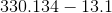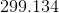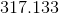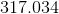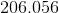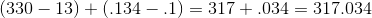# PSAT Math : How to subtract decimals

## Example Questions

### Example Question #34 : Other Decimals

Round the answer to the nearest tenth.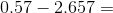Explanation:

0.57 - 2.657 = -2.087

Don't forget the negative sign!

This number then rounds to -2.1.

### Example Question #1 : How To Subtract Decimals

Simplify: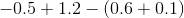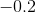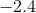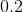Explanation:

Start by simplifying within the parentheses: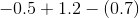Then add/subtract each number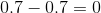When you finish simplifying, the answer is 0.

### Example Question #2 : How To Subtract Decimals

Find the difference.